Author: Oscar Cronquist Article last updated on May 16, 2019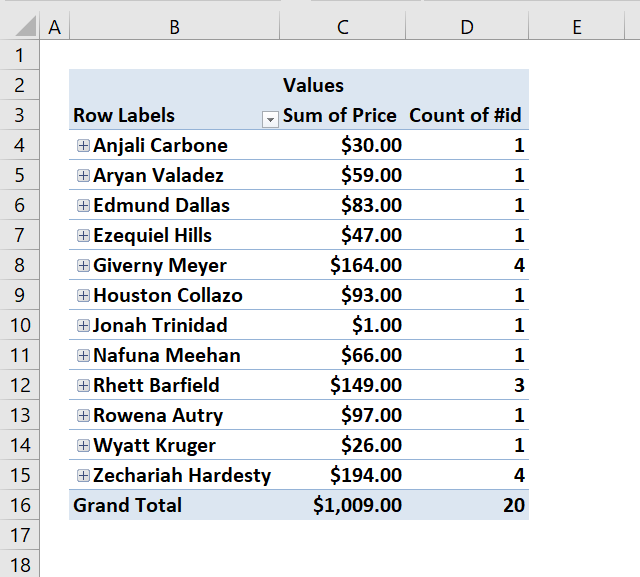I am a convenience store owner that is looking to make a spreadsheet formula. I want this formula to use information from one spreadsheet to auto-populate another spreadsheet on the next tab. I want the date the purchase was made, the consumer, and however many items the consumer purchased to equal one transaction on the other spreadsheet. Your help with this would be greatly appreciated.

Your question is perfect for a Pivot Table. The problem is that you need to refresh the Pivot Table every time you change the source data, however, this can be done automatically with a few lines of event code.

I´ll show you how to set up the pivot table and how to make it auto refresh using VBA. I will also provide an answer for how to accomplish this using only array formulas.

• Create an Excel defined Table.
• Create a Pivot Table.
• How to set up a Pivot Table.
• Use VBA code in your workbook.
• Save your workbook as a macro-enabled workbook.
• Create array formulas and how they work.

Example sheet "Data"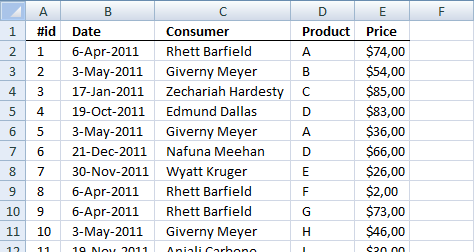###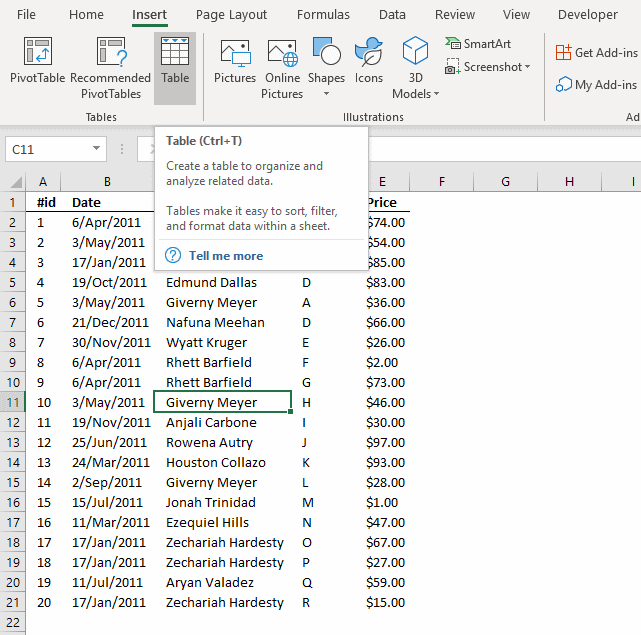Why convert the data set to an Excel defined Table? It expands automatically meaning you don't need to adjust cell references if you add or delete data.

1. Select any cell in your data set.
2. Go to tab "Insert".
3. Press with left mouse button on "Table" button.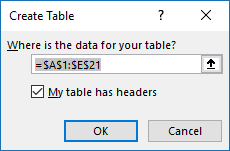4. Press with left mouse button on the checkbox "My Table has headers" if appropriate.
5. Press with left mouse button on OK button.

The image below shows what the Excel defined Table probably will look like.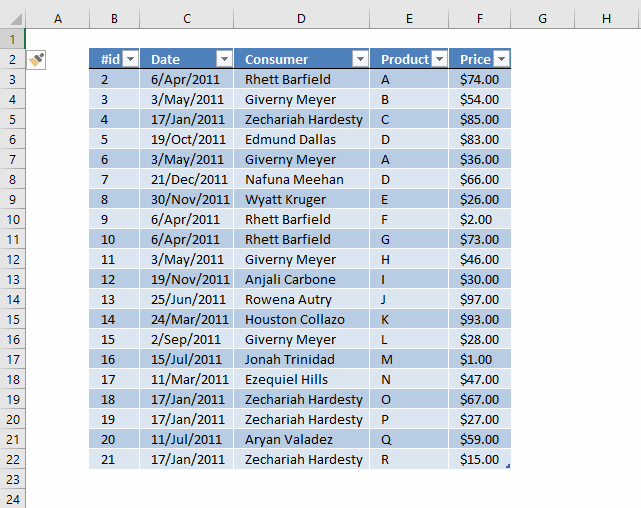### Create a Pivot Table

1. Select all data on Sheet "Data"
2. Go to tab "Insert"
3. Press with left mouse button on "Pivot Table" button
4. Press with left mouse button on "Pivot table" and a dialog box appears.
5. I chose to put the Pivot Table on a new Worksheet.
6. Press and hold with left mouse button on fields in Field List.
7. Drag to different areas below, now release left mouse button.
8. The dates and items go to RowLabels.
9. The Price goes to Values area.

The following animated image shows the above steps in greater detail.

Example sheet "Pivot table"

Below is VBA code to refresh Pivot Table every time the Pivot Table Sheet is activated. You put the VBA code in a worksheet module, instructions below.

```Private Sub Worksheet_Activate()
Sheets("Pivot table").PivotTables("PivotTable1").RefreshTable
End Sub
```

### Where to put the code?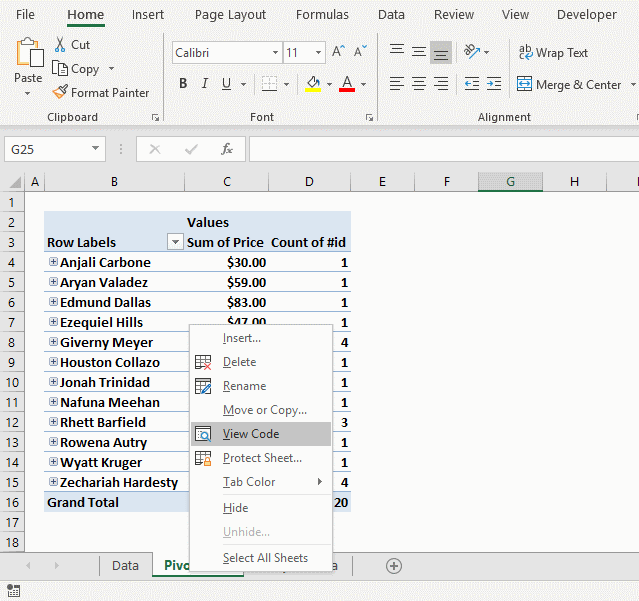1. Press with right mouse button on on sheet name "Pivot table"
2. Press with left mouse button on "View Data".
3. Paste code to the worksheet module.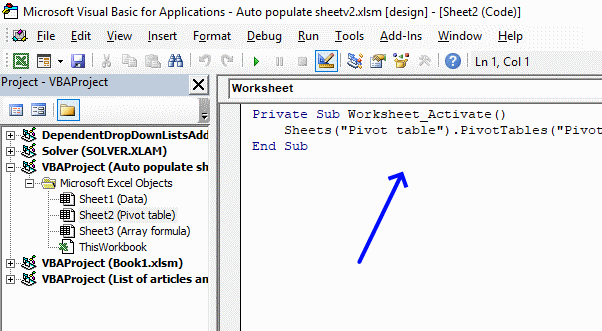Note, save your workbook with the file extension *.xlsm (macro-enabled) in order to keep the code to your workbook.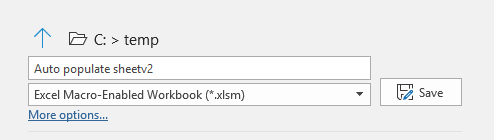### Totals based on Name and Date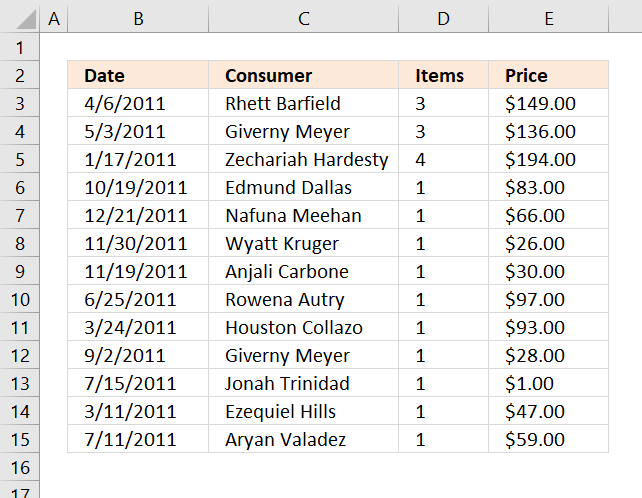The formulas demonstrated here are not necessary if you use a Pivot Table, they simply show how to accomplish the same thing using only formulas. I highly recommend using a Pivot Table.

The image above shows three different formulas, the first formula in cell range B3:C15 extracts unique distinct records based on date and name.

The second formula counts records based on name and date, the third formula adds prices and returns a total also based on date and name.

Array formula in cell B3:

=INDEX(Table2[[Date]:[Consumer]], MATCH(0, COUNTIFS(\$C\$2:C2, Table2[Consumer], \$B\$2:B2, Table2[Date]), 0), COLUMN(A1))

#### How to create an array formula

1. Copy formula above.
2. Select cell B3.
3. Paste formula to cell.
4. Press and hold Ctrl + Shift simultaneously.
5. Press Enter once.
6. Release all keyboard keys.

The formula bar now shows the formula with a beginning and ending curly bracket telling you that you entered the formula successfully. Don't enter the curly brackets yourself, they appear automatically if you did the above steps correctly.

#### How to copy array formula

1. Select cell B3
2. Copy cell. (Short cut keys: Ctrl + c)
3. Select cell range B3:C15
4. Paste to cell range (Short cut keys: Ctrl +v).

#### Explaining formula in cell B3

The array formula contains cell references (structured references) to the Excel defined Table, there is now no need to adjust cell references if the data source expands or shrinks.

You can find an explanation to the formula here: Filter unique distinct records

### Count records based on conditionsFormula in cell D3:

=COUNTIFS(Table2[Consumer], C3, Table2[Date], B3)

Copy cell D3 and paste to cell range D3:D15.

#### Explaining formula in cell D3

This formula counts the number of records based on two conditions, name in the corresponding cell in column C and date in the corresponding cell in column B.

This article explains the formula in greater detail: Count unique distinct records

### Sum amounts based on conditionsFormula in cell E3:

=SUMIFS(Table2[Price], Table2[Consumer], C3, Table2[Date], B3)

This formula is simply a SUMIFS function, read more about it here: How to use the SUMIFS function

### Get the Excel fileAuto-populate-sheet.xlsm